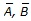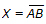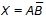# Digital Electronics - Boolean Algebra and Logic Simplification

### Exercise :: Boolean Algebra and Logic Simplification - General Questions

16.

What is the primary motivation for using Boolean algebra to simplify logic expressions?

 A. It may make it easier to understand the overall function of the circuit. B. It may reduce the number of gates. C. It may reduce the number of inputs required. D. all of the above

Answer: Option D

Explanation:

No answer description available for this question. Let us discuss.

17.

How many gates would be required to implement the following Boolean expression after simplification? XY + X(X + Z) + Y(X + Z)

 A. 1 B. 2 C. 4 D. 5

Answer: Option B

Explanation:

No answer description available for this question. Let us discuss.

18.

AC + ABC = AC

 A. True B. False

Answer: Option A

Explanation:

No answer description available for this question. Let us discuss.

19.

Whenare the inputs to a NAND gate, according to De Morgan's theorem, the output expression could be:

 A. X = A + B B.C. X = (A)(B) D.Answer: Option A

Explanation:

No answer description available for this question. Let us discuss.

20.

Which Boolean algebra property allows us to group operands in an expression in any order without affecting the results of the operation [for example, A + B = B + A]?

 A. associative B. commutative C. Boolean D. distributive

Answer: Option B

Explanation:

No answer description available for this question. Let us discuss.

#### Current Affairs 2021

Interview Questions and Answers